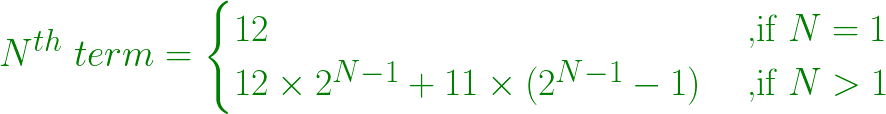Open in App
Not now

# Find the Nth term in series 12, 35, 81, 173, 357, …

• Last Updated : 28 Jul, 2022

Given a number N, the task is to find the Nth term in series 12, 35, 81, 173, 357, …
Example:

```Input: N = 2
Output: 35
2nd term = (12*2) + 11
= 35

Input: N = 5
Output: 357
5th term = (12*(2^4))+11*((2^4)-1)
= 357```

Approach:

• Each and every number is obtained by multiplying the previous number by 2 and the addition of 11 to it.
• Since starting number is 12.

```1st term = 12
2nd term = (12 * 2) / 11 = 35
3rd term = (35 * 2) / 11 = 81
4th term = (81 * 2) / 11 = 173
And, so on....```
•
• In general, Nth number is obtained by formula:•

Below is the implementation of the above approach:

## C++

 `// C++ program to find the Nth term``// in series 12, 35, 81, 173, 357, ...` `#include ``using` `namespace` `std;` `// Function to find Nth term``int` `nthTerm(``int` `N)``{``    ``int` `nth = 0, first_term = 12;` `    ``// Nth term``    ``nth = (first_term * (``pow``(2, N - 1)))``          ``+ 11 * ((``pow``(2, N - 1)) - 1);` `    ``return` `nth;``}` `// Driver Method``int` `main()``{``    ``int` `N = 5;``    ``cout << nthTerm(N) << endl;` `    ``return` `0;``}`

## Java

 `// Java program to find the Nth term``// in series 12, 35, 81, 173, 357, ...``class` `GFG``{` `// Function to find Nth term``static` `int` `nthTerm(``int` `N)``{``    ``int` `nth = ``0``, first_term = ``12``;` `    ``// Nth term``    ``nth = (``int``) ((first_term * (Math.pow(``2``, N - ``1``)))``        ``+ ``11` `* ((Math.pow(``2``, N - ``1``)) - ``1``));` `    ``return` `nth;``}` `// Driver code``public` `static` `void` `main(String[] args)``{``    ``int` `N = ``5``;``    ``System.out.print(nthTerm(N) +``"\n"``);``}``}` `// This code is contributed by Rajput-Ji`

## Python3

 `# Python3 program to find the Nth term``# in series 12, 35, 81, 173, 357, ...` `# Function to find Nth term``def` `nthTerm(N) :` `    ``nth ``=` `0``; first_term ``=` `12``;` `    ``# Nth term``    ``nth ``=` `(first_term ``*` `(``pow``(``2``, N ``-` `1``))) ``+` `\``            ``11` `*` `((``pow``(``2``, N ``-` `1``)) ``-` `1``);` `    ``return` `nth;` `# Driver Method``if` `__name__ ``=``=` `"__main__"` `:` `    ``N ``=` `5``;``    ``print``(nthTerm(N)) ;` `# This code is contributed by AnkitRai01`

## C#

 `// C# program to find the Nth term``// in series 12, 35, 81, 173, 357, ...``using` `System;` `class` `GFG``{` `// Function to find Nth term``static` `int` `nthTerm(``int` `N)``{``    ``int` `nth = 0, first_term = 12;` `    ``// Nth term``    ``nth = (``int``) ((first_term * (Math.Pow(2, N - 1)))``        ``+ 11 * ((Math.Pow(2, N - 1)) - 1));` `    ``return` `nth;``}` `// Driver code``public` `static` `void` `Main(String[] args)``{``    ``int` `N = 5;``    ``Console.Write(nthTerm(N) +``"\n"``);``}``}` `// This code is contributed by PrinciRaj1992`

## Javascript

 ``

Output:

`357`

Time complexity: O(log N) for given input N because using inbuilt pow function

Auxiliary Space: O(1)

My Personal Notes arrow_drop_up